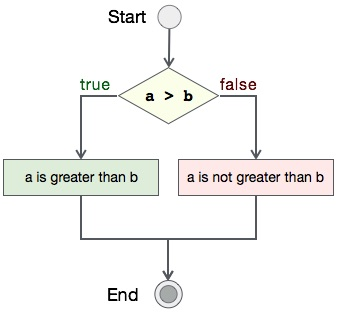# Program to search text in a file

## Algorithm

Let's first see what should be the step-by-step procedure to compare two integers−

```START

Step 1 → Take two integer variables, say A & B

Step 2 → Assign values to variables

Step 3 → Compare variables if A is greater than B

Step 4 → If true print A is greater than B

Step 5 → If false print A is not greater than B

STOP
```

## Flow Diagram

We can draw a flow diagram for this program as given below −## Pseudocode

Let's now see the pseudocode of this algorithm −

```procedure compare(A, B)

IF A is greater than B
DISPLAY "A is greater than B"
ELSE
DISPLAY "A is not greater than B"
END IF

end procedure
```

## Implementation

Assuming we have file with name file_search.txt with this content −

```Tutorials Point originated from the idea that there exists a class of readers who
respond better to online content and prefer to learn new skills at their own pace
from the comforts of their drawing rooms.
```

Now, we shall see the actual implementation of the program −

```#include <stdio.h>
#include <string.h>

int main()
{
FILE *fp;
char word;
char ch;
char *filename = "somefile.txt";
char *search = "to";
int count = 0;
int pos;
int pointer = 0;
int loop;

/*  open for writing */
fp = fopen(filename, "r");

do
{
ch = fscanf(fp, "%s", word);
if(strcmp(word, search) == 0)
{
pos[count] = pointer;
count++;
}
pointer++;
//printf("%s",word);
} while (ch != EOF);

if(count == 0)
else
{
printf("'%s' is found at -> ", search);
for(loop = 0; loop<count; loop++)
{
printf("%d ", pos[loop]);
}
printf("positions.\n");
}

fclose(fp);

return 0;
}
```

## Output

Output of this program should be −

```'to' is found at -> 16 21 positions.
```